# AKNS-hierarchy

(diff) ← Older revision | Latest revision (diff) | Newer revision → (diff)

Ablowitz–Kaup–Newell–Segur hierarchy

An infinite tower of non-linear evolution equations that derives its name from the simplest non-trivial system of equations contained in it, the AKNS-equations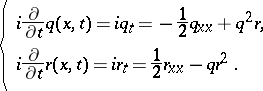(a1)

It were M.J. Ablowitz, D.J. Kaup, A.C. Newell, and H. Segur who showed that the initial value problem of this system of equations (cf. also Differential equation, partial, discontinuous initial (boundary) conditions) could be solved with the inverse scattering transform (cf. also Korteweg–de Vries equation). To get a natural embedding of the AKNS-equations in a larger system, one rewrites (a1) in zero-curvature form as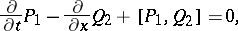(a2)

whereConsider now the following polynomial expressions in the parameter: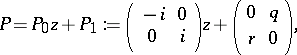(a3)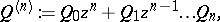where theare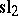-valued functions depending on the variables,, and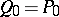and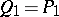. For these data the zero-curvature equations read(a4)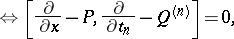which is an infinite tower of equations extending the system (a1). The system (a4) generalizes from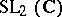to a general simple complex Lie algebra, a regular elementin a Cartan subalgebraofand an element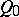in, see [a4] and [a10]. Solutions of the equations (a4) can be obtained by the Zakharov–Shabat dressing method (cf. also Soliton). Namely, consider the function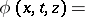(a5)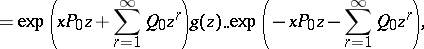with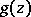belonging to the loop group. If thisfactorizes as, with(a6)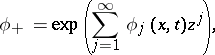then conjugating the trivial connections (cf. also Connections on a manifold)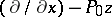and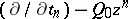withgives connections of the required form: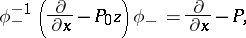(a7)Since flatness is preserved by this procedure, this leads to solutions of (a4). If, as in the AKNS-case, one can take just as well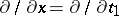.

It was observed by H. Flaschka, Newell and T. Ratiu [a5] that the equations (a4) for the-case could be captured in the system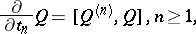(a8)

for the single series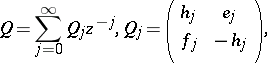(a9)They showed that these equations are commuting Hamiltonian flows (cf. also Hamiltonian system) on the Lie algebra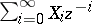,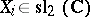, with respect to natural Poisson brackets. Further, they introduced the flux tensor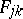by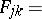(a10)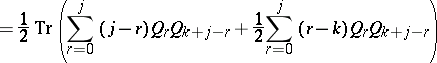and proved the local conservation laws of the system, namely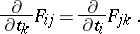(a11)

The left-hand side of (a11) is, in fact, even symmetric under permutations of the indices,,and this property made them introduce a potentialby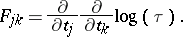(a12)

The equations (a8) are called the Lax equations of the AKNS-hierarchy. As such, the AKNS-hierarchy is a natural reduction of the two-component KP-hierarchy (cf. also KP-equation; [a7]), a fact that enables a description in the Grassmannian of that hierarchy.

It was shown by M.J. Bergvelt and A.P.E. ten Kroode [a1] that it is natural to consider a system of zero-curvature relations (a4) labelled by the root lattice of the Lie algebra, where the operators at different sites of the lattice are linked by Toda-type differential-difference equations. For example, for nearest neighbour sites there holds(a13)

where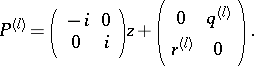This phenomenon is due to the fact that there is a natural lattice group that commutes with the commuting flows corresponding to the parameters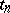.

In the representation-theoretic approach to soliton equations (see [a3], [a8]), the soliton equations occur as the equations describing the group orbit of the highest weight vector. A similar description holds for these combined differential-difference equations. Let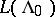be the basic representation of the Kac–Moody Lie algebra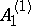(cf. also Kac–Moody algebra). Inone takes the homogeneous Heisenberg algebra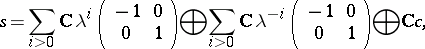whereis the central element ofthat is in the kernel of the projection ofonto the loop algebra of. The-moduledecomposes with respect to the action of the homogeneous Heisenberg algebraas a direct sum of irreducible-moduleslabelled by the root lattice. Thus, one can write each element ofas. The group orbit of the highest weight vector can then be characterized by a set of so-called Hirota bilinear relations for the components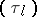. By using the representation theory one constructs a series of elementsin a suitable completion of the Kac–Moody group associated withsuch that the vacuum expectation value of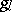is exactly. The Birkhoff decomposition of the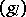in that group then enables one to construct solutions of the lattice zero-curvature equations, [a2]. In particular, the operators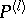from (a13) obtained in this way can be expressed in the componentsby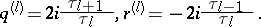(a14)

By using the adjoint action of the Kac–Moody group, Bergvelt and ten Kroode also showed [a2] that the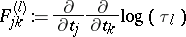(a15)

give exactly the flux tensor from (a10), thus furnishing a representation-theoretic basis for the results in [a5].

A geometric way to look at-functions, see e.g. [a9], is to consider a homogeneous space over the relevant loop group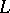, a holomorphic line bundleover this space and its pull-back over the corresponding central extension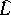of. If this last line bundle has a global holomorphic section, then-functions measure the failure of equivariance of partial liftings fromtowith respect to this section. With this point of view, one can also arrive at the formulas in (a14) by lifting the discrete group of transformations that commute with the flows from (a6) appropriately, see [a11].

An important class of equations associated with the AKNS-hierarchy are the so-called stationary AKNS-equations. These are the differential equations for the functionsandfrom the first-order differential operator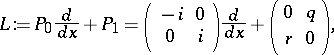resulting from the existence of a-matrix-valued differential operator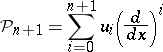that commutes with. Such a pair is naturally associated with a hyper-elliptic curve of genusand that is why one callsan algebro-geometric AKNS-potential. The elliptic algebro-geometric AKNS-potentials have been characterized in [a6]. They correspond exactly to the potentials for which the equation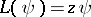has a meromorphic fundamental system of solutions with respect tofor all values of the spectral parameter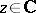.

How to Cite This Entry:
AKNS-hierarchy. Encyclopedia of Mathematics. URL: http://encyclopediaofmath.org/index.php?title=AKNS-hierarchy&oldid=16838
This article was adapted from an original article by G.F. Helminck (originator), which appeared in Encyclopedia of Mathematics - ISBN 1402006098. See original article